# Frank Solutions for Chapter 11B Alkanes Class 10 Chemistry ICSE

1. Which organic compound is indicated by marsh gas and fire damp ?

Methane is indicated by marsh gas and fire damp.

2. (a) How is methane gas prepared in laboratory ?
(b) How is the gas collected ?
(c) Why is soda lime used not only NaOH ?

(a) Mixture of sodium ethanoate and soda lime is heated in a hard glass tube.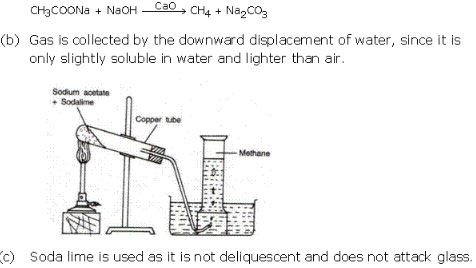3. (a) How is ethane prepared by Wurtz reaction ?
(b) How can we prepare ethane by reduction of a halogen compound ?

(a) When methyl bromide or methyl iodide and sodium are heated in presence of dry ether, ethane is formed.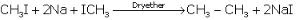(b) By reduction of Ethyl iodide using Zn + Cu couple in alcohol, ethane is formed.4. (a) What are products obtained when methane reacts with chlorine in diffused sunlight ?
(b) Write the equation for above reactions.
(c) Name and define the type of above reaction .

(a) The products obtained when methane reacts with chlorine in diffused sunlight are Chloromethane, Dichloromethane, Trichloromethane and Tetrachoromethane.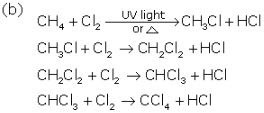(c) The above reaction is substitution reaction. Here the substitution of alkanes with chlorine takes place hence it is called chlorination.
the reactions in which the hydrogen of the alkane molecule is replaced by another atom or group of atoms resulting in the formation of the derivative of that hydrocarbon are called substitution reactions.
Substitution by halogen atom is called halogenations.

5. Give two uses each of:
(i) Methane
(ii) Ethane

(i) Uses of Methane

• As a domestic fuel in the form of natural gas
• In the manufacture of methanol and hydrogen.
(ii) Uses of Ethane
• As a fuel, it has high calorific value than methane. Liquefied ethane is also used as a fuel.
• In the preparation of ethanol, acetaldehyde and acetic add which find use in paints, varnishes, adhesive, plastic etc.

6. Write the equations of chlorination of ethane.

7. How will you bring about following conversions :
(a) Methane to methanol
(b) Ethane to ethanol (acetaldehyde)
(c) Methane to methanoic acid

8. Write the structural formulae of isomers of butane.

9. Under what conditions does ethane get converted to
(a) Ethyl alcohol
(b) acetic acid

(a) Ethane is mixed oxygen and is passed through hot copper tube ; it gets oxidized to ethyl alcohol.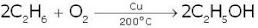(b) Ethane is first converted into ethyl alcohol by passing over hot copper tube, then further oxidation with acidified potassium dichromate yield aldehyde and carboxylic acids.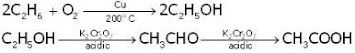10. What are the main sources of alkanes and why they are known as saturated hydrocarbons ?

The main sources of alkanes are natural gas and petroleum.
Alkanes are known as saturated hydrocarbons because the carbon atoms in their molecules are bonded to each other by single covalent bond. Each carbon atom is again bonded to hydrogen atom.

11. What is greenhouse gas? What is the contribution of methane in causing greenhouse effect?

Some components of the atmosphere such as water vapour, carbon dioxide, nitrous oxide and methane absorb heat energy reflected by the earth's surface and hence increase the average atmospheric temperature. These gases are called greenhouse gases.
Methane contributes to the greenhouse effect to an extent of 19%, and the concentration of methane has been increasing at the rate of 1.5% per year.

12. What are the products obtained by heating ethane in :
(a) Excess of air
(b) Absence of air
(c) Air in presence of copper and 120 atm pressure
(d) Air with molybdenum oxide at 100 atm pressure.

(a) Ethane reacts in excess of air to give carbon dioxide and water.(b) Ethane reacts in absence of air to give carbon black.(c) Ethane reacts in presence of copper and 120 atm pressure to give ethanol(d) Ethane reacts with molybdenum oxide at 100 atm pressure to give ethanol .13. How many covalent bonds are present in ethane ?